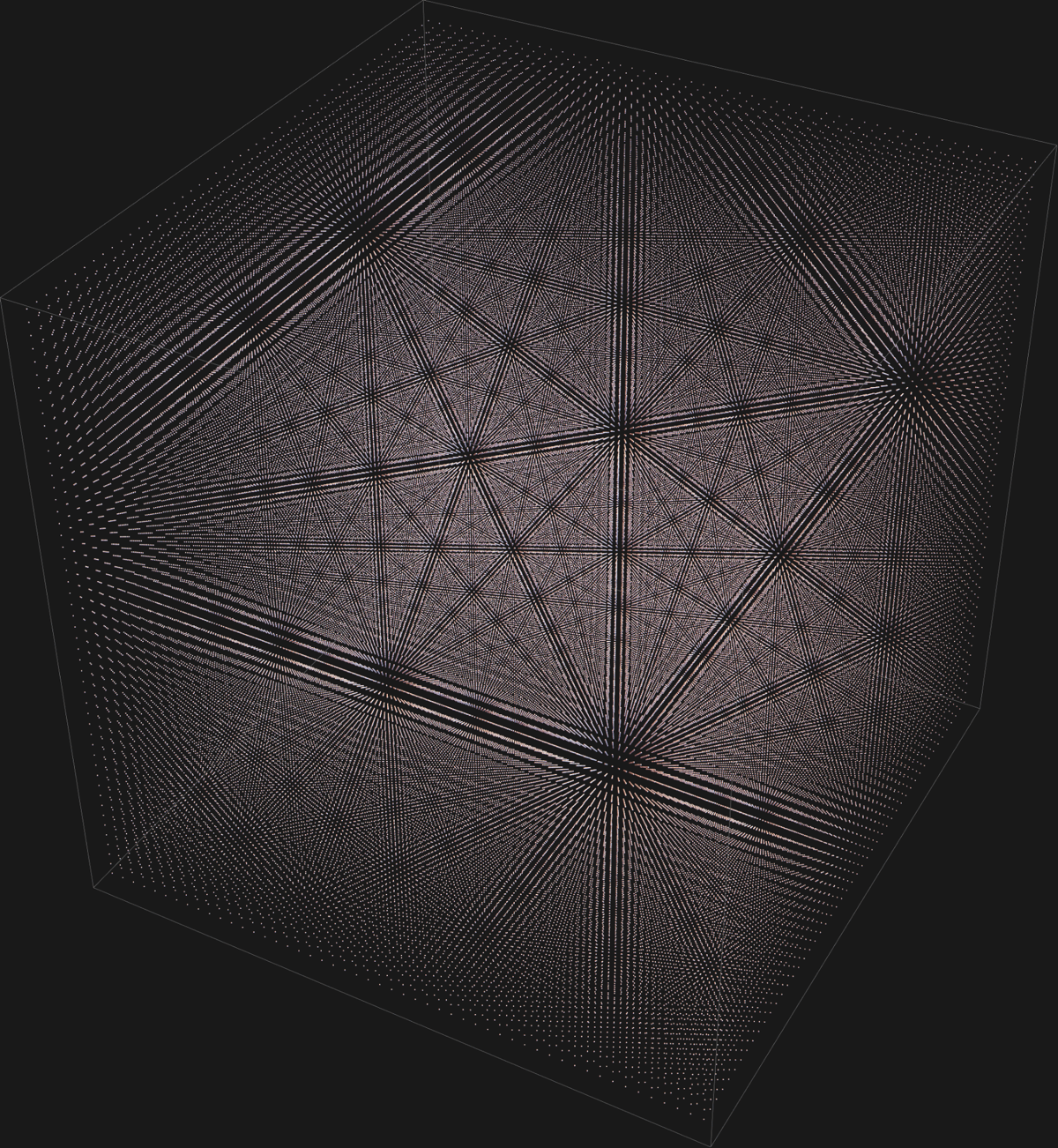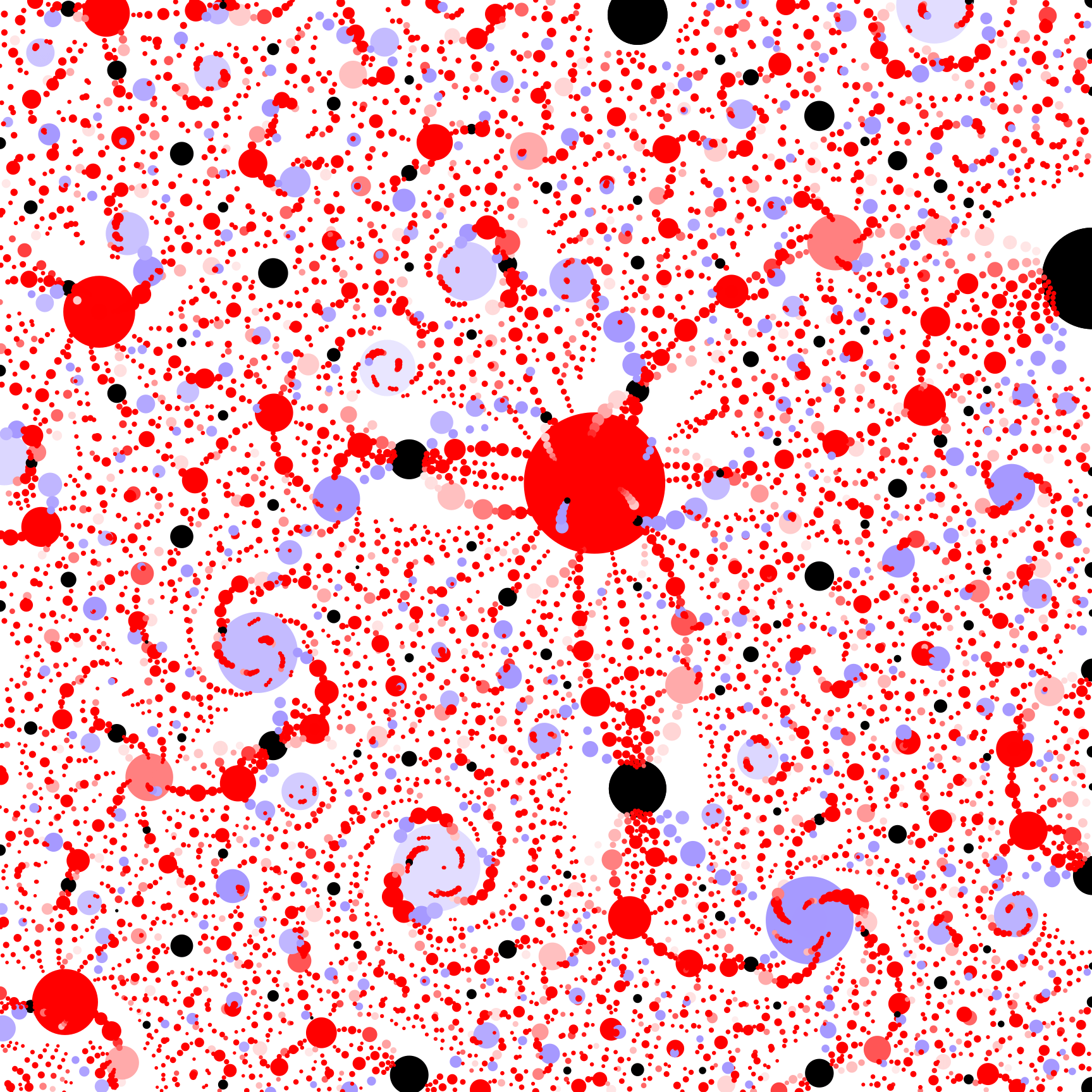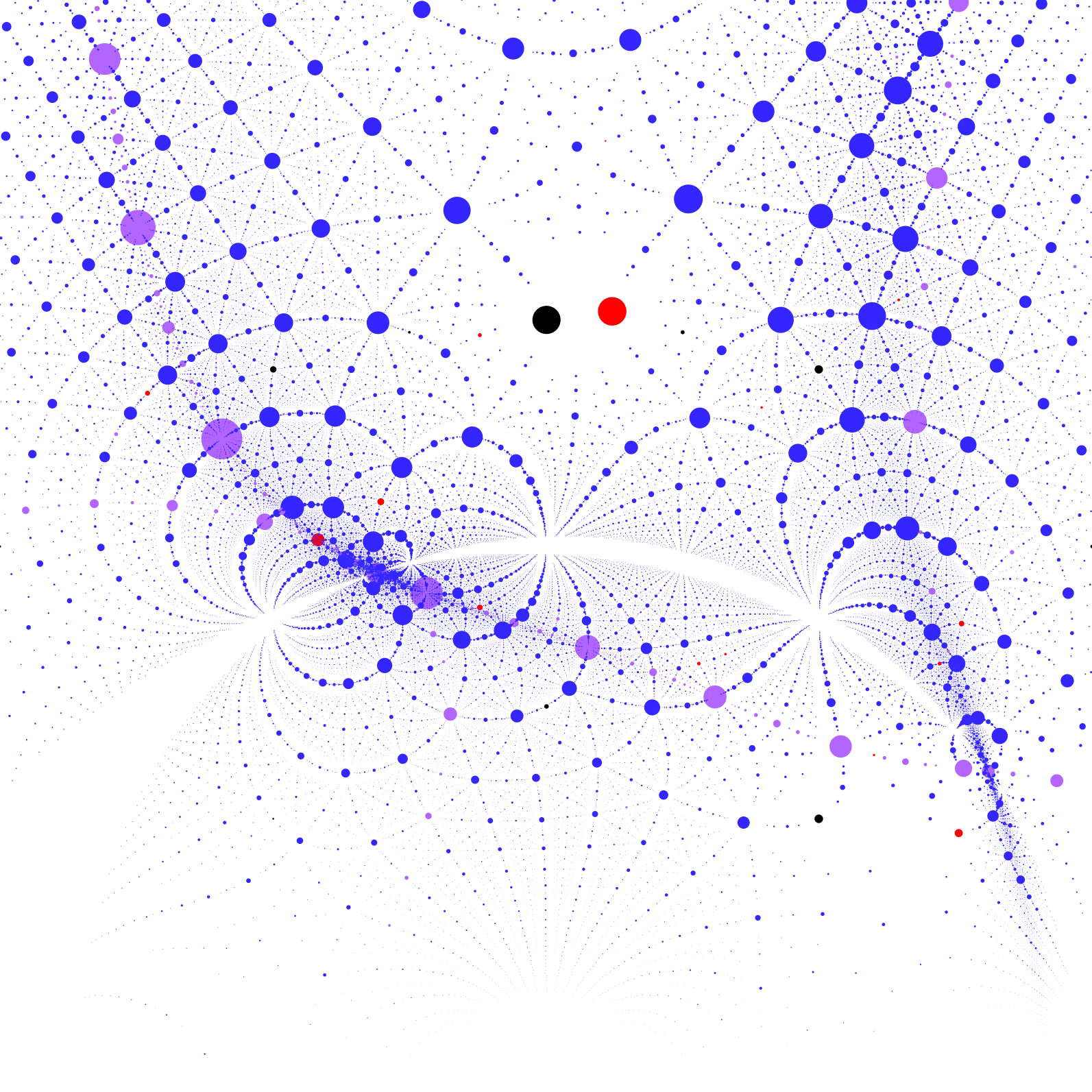# Visualizing Algebraic Numbers

Date started: October 2019
Leads: Pierre Arnoux, Edmund HarrissKatherine StangeSteve Trettel

###Abstract

The algebraic numbers are dense in the complex plane, so drawing a dot for each will result in a black canvas.  However, by clever choices of subsets and radii, such pictures can reveal stunning structure among the algebraic numbers.  For example, quadratic irrationals accumulate along hyperbolic geodesics of the upper half plane, and exhibit a Diophantine approximation gap principle governed by discriminant.  Cubics appear more chaotic until restrictions are placed on their coefficients, when they reveal striking dynamics mimicking spiral galaxies. This project uses such visualizations both for research (with connections to Diophantine approximation, Lehmer’s conjecture, etc.), and to create art.

### MediaThe complex cubic numbers with negative real root (red) and some with positive real root (blue). (Image: Edmund Harriss)Algebraic Abstract Expressionism (detail of the first). (Image: Edmund Harriss)Quintic starscape, the roots of a x^5 + b x^3 + c x^2 + b x + a. (Image: Edmund Harriss)

In the space of coefficients, the integer polynomials$ax^2+bx+c$ realize the cubic lattice in$\mathbb{R}^3$ (first picture below), and modulo scaling gives a countable set in$\mathbb{RP}^2$ (second picture below).

The map assigning a polynomial to its roots is a homeomorphism onto the upper half plane when restricted to those with negative discriminant (green region in second picture below), realizing (projective) symmetries of the cubic lattice as symmetries of the set of quadratic numbers.